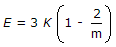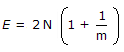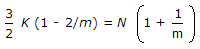# Civil Engineering - Theory of Structures - Discussion

### Discussion :: Theory of Structures - Section 1 (Q.No.26)

26.

If E, N, K and 1/m are modulus of elasticity, modulus of rigidity. Bulk modulus and Poisson ratio of the material, the following relationship holds good

 [A].[B].[C].[D]. all the above.

Explanation:

No answer description available for this question.

 Babu said: (Jun 27, 2017) Relation between E and C: E = 2C [1+ μ], Relation between E and K: 3K (1-2μ), Relation between E, C and K: E=9KC/ (3K+C).

 Hemant Maher said: (Aug 17, 2017) Here, 1/m=μ